Courses

# Propositional & First Order Logic MCQ - 1

## 20 Questions MCQ Test GATE Computer Science Engineering(CSE) 2022 Mock Test Series | Propositional & First Order Logic MCQ - 1

Description
This mock test of Propositional & First Order Logic MCQ - 1 for Computer Science Engineering (CSE) helps you for every Computer Science Engineering (CSE) entrance exam. This contains 20 Multiple Choice Questions for Computer Science Engineering (CSE) Propositional & First Order Logic MCQ - 1 (mcq) to study with solutions a complete question bank. The solved questions answers in this Propositional & First Order Logic MCQ - 1 quiz give you a good mix of easy questions and tough questions. Computer Science Engineering (CSE) students definitely take this Propositional & First Order Logic MCQ - 1 exercise for a better result in the exam. You can find other Propositional & First Order Logic MCQ - 1 extra questions, long questions & short questions for Computer Science Engineering (CSE) on EduRev as well by searching above.
QUESTION: 1

### What is the logical translation of the following statement? "None of my friends are perfect."

Solution:

F(x) ==> x is my friend
P(x) ==> x is perfect

D is the correct answer.

A. There exist some friends which are not perfect

B. There are some people who are not my friend and are perfect

C. There exist some people who are not my friend and are not perfect.

D. There doesn't exist any person who is my friend and perfect

QUESTION: 2

### Let # be a binary operator defined as X # Y = X′ + Y′ where X and Y are Boolean variables. Consider the following two statements. S1: (P # Q) # R = P # (Q # R) S2: Q # R = R # Q Which of the following is/are true for the Boolean variables P, Q and R?

Solution:

S2 is true, as X' + Y' = Y' + X'

S1 is false.
Let P = 1, Q = 1, R = 0, we get different results
(P # Q) # R = (P' + Q')' + R' = (0 + 0)' + 1 = 1 + 1 = 1
P # (Q # R) = P' + (Q' + R')' = 0 + (0 + 1)' = 0 + 0 = 0

QUESTION: 3

### What is the correct translation of the following statement into mathematical logic? “Some real numbers are rational”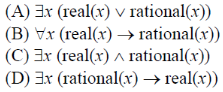Solution:
QUESTION: 4

Which one of the following options is CORRECT given three positive integers x, y and z, and a predicate?

P(x) = ¬(x=1)∧∀y(∃z(x=y*z)⇒(y=x)∨(y=1))

Solution: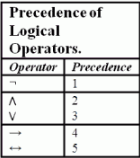So the predicate is evaluated asP(x) = (¬(x=1))∧(∀y(∃z(x=y*z)⇒((y=x)∨(y=1))) P(x) being true means x ≠ 1 and For all y if there exists a z such that x = y*z then y must be x (i.e. z=1) or y must be 1 (i.e. z=x) It means that x have only two factors first is 1 and second is x itself. This predicate defines the prime number.

QUESTION: 5

Suppose the predicate F(x, y, t) is used to represent the statement that person x can fool person y at time t. which one of the statements below expresses best the meaning of the formula ∀x∃y∃t(¬F(x, y, t))?

Solution:

∀ x ∃ y ∃ t(¬ F(x,y,t)) => ∀ x ¬(∀ y ∀ t F(x,y,t))

QUESTION: 6

Which one of the following is the most appropriate logical formula to represent the statement? "Gold and silver ornaments are precious". The following notations are used: G(x): x is a gold ornament S(x): x is a silver ornament P(x): x is precious

Solution:

=> This statement can be expressed as => For all X, x can be either gold or silver then the ornament X is precious => For all X, (G(X) v S(x)) => P(X).

QUESTION: 7

In propositional logic P ↔ Q is equivalent to (Where ~ denotes NOT):

Solution:

P ↔ Q = (P → Q) ∧ (Q → P)
P ↔ Q = (~P ∨ Q) ∧ (~Q v P)
So, option (B) is correct.

QUESTION: 8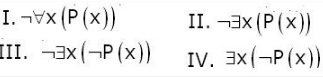Q. Which of the above two are equivalent?

Solution:

According to negation property of universal qualifier and existential quantifier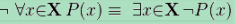QUESTION: 9

Let fsa and pda be two predicates such that fsa(x) means x is a finite state automaton, and pda(y) means that y is a pushdown automaton. Let equivalent be another predicate such that equivalent (a, b) means a and b are equivalent. Which of the following first order logic statements represents the following: Each finite state automaton has an equivalent pushdown automaton.

Solution:

Considering each option :
(A) If everything is a FSA, then there exists an equivalent PDA for everything.
(B) It is not the case that for all y if there exist a FSA then it has an equivalent PDA.
(C) Everything is a FSA and has an equivalent PDA.
(D) Everything is a PDA and has exist an equivalent FSA.
Thus, option (A) is correct.

Please comment below if you find anything wrong in the above post.

QUESTION: 10

P and Q are two propositions. Which of the following logical expressions are equivalent?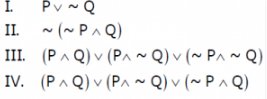Solution:

I and II are same by Demorgan's law The IIIrd can be simplified to I.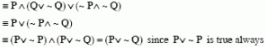QUESTION: 11

Let Graph(x) be a predicate which denotes that x is a graph. Let Connected(x) be a predicate which denotes that x is connected. Which of the following first order logic sentences DOES NOT represent the statement: “Not every graph is connected”?

Solution:

Option A and option C are same G(x)-->C(x) can also be written as ~G(x) or C(x), and they are the correct representation. Option C says :There exists a graph and graph is not connected ,which is equivalent to given sentence. Option D: Every x which is graph is not connected. Option D is correct choice

QUESTION: 12

Which of the following is TRUE about formulae in Conjunctive Normal Form?

Solution:

We can easily prove that for any formula, there is a truth assignment for which at least half the clauses evaluate to true . Proof : Consider an arbitrary truth assignment. For each of its clause ‘j’ , introduce a random variable. Xj = 1 if clause ‘j’ is satisfied Xj = 0 otherwise Then, X = summation of (j * Xj) is the number of satisfied clauses. Given any clause ’c’ , it is unsatisfied only if all of its ‘k’ constituent literals evaluates to false as they are joined by OR operator. Now, because each literal within a clause has a 1/2 chance of evaluating to true independently of any of the truth value of any of the other literals, the probability that they are all false is (1 / 2)k . Thus, the probability that ‘c’ is satisfied = 1 − (1 / 2)k So, E(Xj) = 1 * (1 / 2)k = (1 / 2)k Therefore, E(Xj) >= 1/2 Summation on both sides to get E(X). Therefore, we have E(X) = summation of (j * Xj) >= m/2 where ‘m’ is the number of clauses. E(X) represents expected number of satisfied clauses. Thus, there must exist an assignment that satisfies at least half of the clauses. Please comment below if you find anything wrong in the above post.

QUESTION: 13

The following propositional statement is (P → (Q v R)) → ((P ^ Q) → R)

Solution: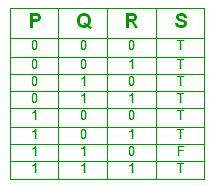A formula is satisfiable if there is atleast one assignment for which it is true. Clearly this formula is satisfiable as there are 7 assignments for which it is true.
A formula is valid if it is true for all assignments, which is not the case here.
Thus, option (B) is correct.

QUESTION: 14

Which one of the following Boolean expressions is NOT a tautology?

Solution:

(A) a -> b means if ‘a’ is true then ‘b’ is also true. Now, ‘b’ is true. Therefore, ‘c’ is also true. => Using transitivity rule , a -> c
(B) a <-> c is true if both ‘a’ and ‘c’ have same values. Let ‘a’, ‘b’ and c’ be false. Expression 'a and b' is false and expression 'not b' is true. RHS of the given equation should be true. But it evaluates to be false. Therefore, contradiction is there.
Option (B) is not a tautology.
(C) Let ‘a’ and ‘c’ be false. ’b’ be true a and b and c is false c or a is false
(D) Let ‘b’ be true. b -> a means if ‘b’ is true then ‘a’ is also true. Therefore, ‘a’ and ‘b’ both evaluates to be true.

Thus, option (B) is correct.

Please comment below if you find anything wrong in the above post.

QUESTION: 15

The CORRECT formula for the sentence, “not all rainy days are cold” is

Solution:

(A) Note that (p ∧ ~q) ≡ ~(p -> q). So it means rainy day to cold implication is false for all days. Which means non-rainy days are cold. (B) For all days, if day is not rainy, then it is cold [Non-Rainy days are cold] (C) There exist some days for which not rainy implies cold. [Some non-rainy days are cold] (D) Note that (p ∧ ~q) ≡ ~(p -> q). So it means rainy day to cold implication is false for some days. Which means not all rainy days are cold.

QUESTION: 16

Which one of the first order predicate calculus statements given below correctly express the following English statement?

Tigers and lions attack if they are hungry or threatened.

Solution:

The statement "Tigers and lions attack if they are hungry or threatened" means that if an animal is either tiger or lion, then if it is hungry or threatened, it will attack. So option (D) is correct. Don't get confused by "and" between tigers and lions in the statement. This "and" doesn't mean that we will write "tiger(x) ∧ lion(x) ", because that would have meant that an animal is both tiger and lion, which is not what we want.

QUESTION: 17

Consider the following propositional statements:
P1 : ((A ∧ B) → C)) ≡ ((A → C) ∧ (B → C))
P2 : ((A ∨ B) → C)) ≡ ((A → C) ∨ (B → C))
which one of the following is true?

Solution:

The easiest way to solve this question by creating truth tables for the expressions given. Note that P1 will be a tautology if truth table for left expression is exactly same as truth table for right expression. Same holds for P2 also.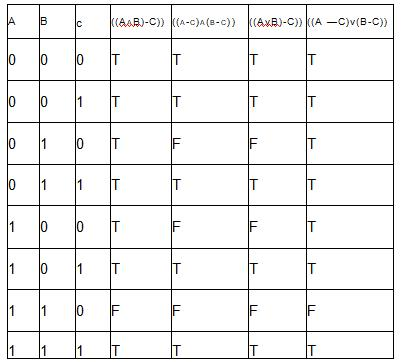So as we see from table, none of the P1 or P2 are tautologies, so option (D) is correct.

QUESTION: 18

A logical binary relation □ ,is defined as follows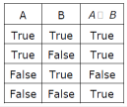Q. Let ~ be the unary negation (NOT) operator, with higher precedence than □. Which one of the following is equivalent to A∧B ?

Solution:

In A∧B, we have 3 entries as False, and one as True. In table, it is opposite case, so we have to negate A □ B, moreover, we want True only when both A and B are true, so in 3rd entry (which becomes true after negation), we want both true, so we have to negate A also. So A ∧ B ≡ ~(~A □ B), so option (D) is correct.

QUESTION: 19

Let P, Q and R be three atomic prepositional assertions. Let X denote (P v Q) → R and Y denote (P → R) v (Q → R). Which one of the following is a tautology?

Solution:

Answer : [ B ]
X = (P ⋁ Q) → R
= ~(P ⋁ Q) ⋁ R

= (~P ⋀ ~Q) ⋁ R

= (~P ⋁ R) ⋀ (~Q ⋁ R)

= (P → R) ⋀ (Q → R)

X → Y is true as (A ⋀ B) → (A ⋁ B) is always TRUE but reverse implication is not always true.

QUESTION: 20

What is the first order predicate calculus statement equivalent to the following?

Every teacher is liked by some student

Solution: